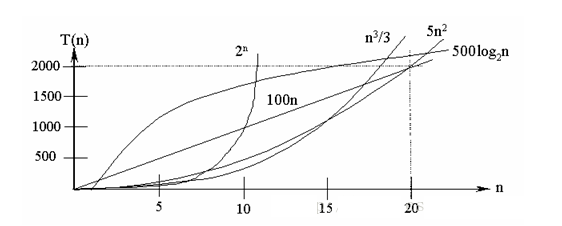### 空间复杂度

#### 概念

Space Complexity,对一个算法在运行过程中临时占用存储度空间的度量，记做S(n)=O(f(n));

int a = 1;
a++;
int b = 2;
b+=1;
int c = a + b;

int n = 10;
int [] arr = new int[n];

int mi = 2;
int m = (int)Math.pow(n,mi);
int [] arr1 = new int[m];


$$S(n)=O(1)$$
arr 数组分配的空间和它的初始长度n 相关，所以它的空间复杂度我们记做：
$$S(n) = O(n);$$
arr1 数组分配的空间和它的初始长度m 相关，而m 是n的mi次方即: m = n^mi

$$S(n) = O(n^2)$$

### 时间复杂度

#### 概念

Time Complexity, 对一个算法运行时所需要消耗的时间的度量，记做：T(n) = O(f(n));

#### O(1)

int count;
int i = 1;
int j = 2;
int k = 3;
count = i + j + k;


$$T(n)=O(1)$$

#### O(log3n)

int n = 100;
int i = 1;
while(i<=n){
i=3*i;
}


$$T(n) = O(log_3n)$$

#### O(n)

int n = 1000;
for(int i = 0;i<n;i++){
System.out.println(i);
}


$$T(n)=O(n)$$

#### O(nlogN)

int n = 1000;
int j;
for(int i = 0;i<n;i++){
j = 1;
while(j<n){
j = 2*j;
}
}


$$T(n)= O(nlogN)$$

#### O(n^k)

int n = 100;
for(int i = 0;i<n;i++){

for(int j = 0;j<n;j++){

for(int k = 0;k<n;k++){

}
}
}


$$T(n) = O(n^3)$$

#### 常见时间复杂度函数图2^n > (n^3)/3 > 5n^2 > 500log(2)(n) > 100n

$$O(2n) > O(n3)>O(n^2)>O(nlog_2n)>O(n)>O(log_2n)>O(1)$$